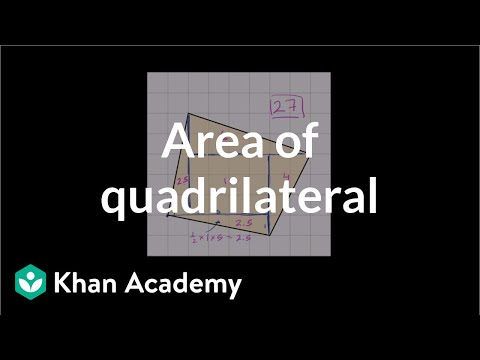Video

# Drawing a quadrilateral on the coordinate plane example (Full video)

Description: Sal draws a quadrilateral on the coordinate plane using the coordinates of its vertices. Created by Sal Khan. Use the line segments below to create a quadrilateral, so a four-sided figure, with vertices at the following points. So they give us four coordinates right over here. So 8, negative 7 Is right over here.

### Other videos you might be interested in### Area of a parallelogram (Full video)